# 14   Dynamics of TCP Reno¶

In this chapter we introduce, first and foremost, the possibility that there are other TCP connections out there competing with us for throughput. In 6.3   Linear Bottlenecks (and in 13.7   TCP and Bottleneck Link Utilization) we looked at the performance of TCP through an uncontested bottleneck; now we allow for competition.

We also look more carefully at the long-term behavior of TCP Reno (and Reno-like) connections, as the value of cwnd increases and decreases according to the TCP sawtooth. In particular we analyze the average cwnd; recall that the average cwnd divided by the RTT is the connection’s average throughput (we momentarily ignore here the fact that RTT is not constant, but the error this introduces is usually small).

A few of the ideas presented here apply as well to non-Reno connections as well. Some non-Reno TCP alternatives are presented in the following chapter; the section on TCP Friendliness below addresses how to extend TCP Reno’s competitive behavior even to UDP.

We also consider some router-based mechanisms such as RED and ECN that take advantage of TCP Reno’s behavior to provide better overall performance.

The chapter closes with a summary of the central real-world performance problems faced by TCP today; this then serves to introduce the proposed TCP fixes in the following chapter.

## 14.1   A First Look At Queuing¶

In what order do we transmit the packets in a router’s outbound-interface queue? The conventional answer is in the order of arrival; technically, this is FIFO (First-In, First-Out) queuing. What happens to a packet that arrives at a router whose queue for the desired outbound interface is full? The conventional answer is that it is dropped; technically, this is known as tail-drop.

While FIFO tail-drop remains very important, there are alternatives. In an admittedly entirely different context (the IPv6 equivalent of ARP), RFC 4681 states, “When a queue overflows, the new arrival SHOULD replace the oldest entry.” This might be called “head drop”; it is not used for router queues.

An alternative drop-policy mechanism that has been considered for router queues is random drop. Under this policy, if a packet arrives but the destination queue is full, with N packets waiting, then one of the N+1 packets in all – the N waiting plus the new arrival – is chosen at random for dropping. The most recent arrival has thus a very good chance of gaining an initial place in the queue, but also a reasonable chance of being dropped later on.

While random drop is seldom if ever put to production use its original form, it does resolve a peculiar synchronization problem related to TCP’s natural periodicity that can lead to starvation for one connection. This situation – known as phase effects – will be revisited in 16.3.4   Phase Effects. Mathematically, random-drop queuing is sometimes more tractable than tail-drop because a packet’s loss probability has little dependence on arrival-time race conditions with other packets.

### 14.1.1   Priority Queuing¶

A quite different alternative to FIFO is priority queuing. We will consider this in more detail in 17.3   Priority Queuing, but the basic idea is straightforward: whenever the router is ready to send the next packet, it looks first to see if it has any higher-priority packets to send; lower-priority packets are sent only when there is no waiting higher-priority traffic. This can, of course, lead to complete starvation for the lower-priority traffic, but often there are bandwidth constraints on the higher-priority traffic (eg that it amounts to less than 10% of the total available bandwidth) such that starvation does not occur.

In an environment of mixed real-time and bulk traffic, it is natural to use priority queuing to give the real-time traffic priority service, by assignment of such traffic to the higher-priority queue. This works quite well as long as, say, the real-time traffic is less than some fixed fraction of the total; we will return to this in 18   Quality of Service.

## 14.3   TCP Fairness with Synchronized Losses¶

This brings us to the question of just what is a “fair” division of bandwidth. A starting place is to assume that “fair” means “equal”, though, as we shall see below, the question does not end there.

For the moment, consider again two competing TCP connections: Connection 1 (in blue) from A to C and Connection 2 (in green) from B to D, through the same bottleneck router R, and with the same RTT. The router R will use tail-drop queuing.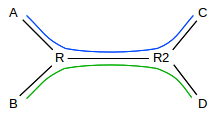The layout illustrated here, with the shared link somewhere in the middle of each path, is sometimes known as the dumbbell topology.

For the time being, we will also continue to assume the synchronized-loss hypothesis: that in any one RTT either both connections experience a loss or neither does. (This assumption is suspect; we explore it further in 14.3.3   TCP RTT bias and in 16.3   Two TCP Senders Competing). This was the model reviewed previously in 13.1.1.1   A first look at fairness; we argued there that in any RTT without a loss, the expression (cwnd1 - cwnd2) remained the same (both cwnds incremented by 1), while in any RTT with a loss the expression (cwnd1 - cwnd2) decreased by a factor of 2 (both cwnds decreased by factors of 2).

Here is a graphical version of the same argument, as originally introduced in [CJ89]. We plot cwnd1 on the x-axis and cwnd2 on the y-axis. An additive increase of both (in equal amounts) moves the point (x,y) = (cwnd1,cwnd2) along the line parallel to the 45° line y=x; equal multiplicative decreases of both moves the point (x,y) along a line straight back towards the origin. If the maximum network capacity is Max, then a loss occurs whenever x+y exceeds Max, that is, the point (x,y) crosses the line x+y=Max.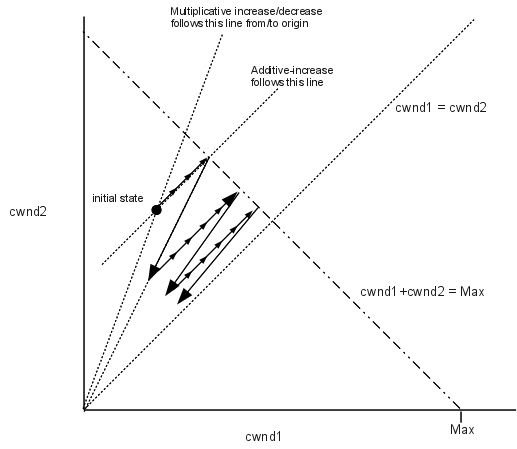Beginning at the initial state, additive increase moves the state at a 45° angle up to the line x+y=Max, in small increments denoted by the small arrowheads. At this point a loss would occur, and the state jumps back halfway towards the origin. The state then moves at 45° incrementally back to the line x+y=Max, and continues to zigzag slowly towards the equal-shares line y=x.

Any attempt to increase cwnd faster than linear will mean that the increase phase is not parallel to the line y=x, but in fact veers away from it. This will slow down the process of convergence to equal shares.

Finally, here is a timeline version of the argument. We will assume that the A–C path capacity, the B–D path capacity and R’s queue size all add up to 24 packets, and that in any RTT in which cwnd1 + cwnd2 > 24, both connections experience a packet loss. We also assume that, initially, the first connection has cwnd=20, and the second has cwnd=1.

T A–C B–D
0 20 1
1 21 2
2 22 3 total cwnd is 25; packet loss
3 11 1
4 12 2
5 13 3
6 14 4
7 15 5
8 16 6
9 17 7
10 18 8 second packet loss
11 9 4
12 10 5
13 11 6
14 12 7
15 13 8
16 14 9
17 15 10 third packet loss
18 7 5
19 8 6
20 9 7
21 10 8
22 11 9
23 12 10
24 13 11
25 14 12 fourth loss
26 7 6 cwnds are quite close
...
32 13 12 loss
33 6 6 cwnds are equal

So far, fairness seems to be winning.

### 14.3.1   Example 2: Faster additive increase¶

Here is the same kind of timeline – again with the synchronized-loss hypothesis – but with the additive-increase increment changed from 1 to 2 for the B–D connection (but not for A–C); both connections start with cwnd=1. Again, we assume a loss occurs when cwnd1 + cwnd2 > 24

T A–C B–D
0 1 1
1 2 3
2 3 5
3 4 7
4 5 9
5 6 11
6 7 13
7 8 15
8 9 17 first packet loss
9 4 8
10 5 10
11 6 12
12 7 14
13 8 16
14 9 18 second loss
15 4 9 essentially where we were at T=9

The effect here is that the second connection’s average cwnd, and thus its throughput, is double that of the first connection. Thus, changes to the additive-increase increment lead to very significant changes in fairness.

### 14.3.2   Example 3: Longer RTT¶

For the next example, we will return to standard TCP Reno, with an increase increment of 1. But here we assume that the RTT of the A–C connection is double that of the B–D connection, perhaps because of additional delay in the A–R link. The longer RTT means that the first connection sends packet flights only when T is even. Here is the timeline, where we allow the first connection a hefty head-start. As before, we assume a loss occurs when cwnd1 + cwnd2 > 24.

T A–C B–D
0 20 1
1 2
2 21 3
3 4
4 22 5 first loss
5 2
6 11 3
7 4
8 12 5
9 6
10 13 7
11 8
12 14 9
13 10
14 15 11 second loss
15 5
16 7 6
17 7
18 8 8 B–D has caught up
20 9 10 from here on only even values for T shown
22 10 12
24 11 14 third loss
26 5 8 B–D is now ahead
28 6 10
30 7 12
32 8 14
34 9 16 fourth loss
35 8
36 4 9
38 5 11
40 6 13
42 7 15
44 8 17 fifth loss
45 8
46 4 9 exactly where we were at T=36

The interval 36≤T<46 represents the steady state here; the first connection’s average cwnd is 6 while the second connection’s average is (8+9+...+16+17)/10 = 12.5. Worse, the first connection sends a windowful only half as often. In the interval 36≤T<46 the first connection sends 4+5+6+7+8 = 30 packets; the second connection sends 125. The cost of the first connection’s longer RTT is quadratic; in general, as we argue more formally below, if the first connection has RTT = 𝜆 > 1 relative to the second’s, then its bandwidth will be reduced by a factor of 1/𝜆2.

Is this fair?

Early thinking was that there was something to fix here; see [F91] and [FJ92], §3.3 where the Constant-Rate window-increase algorithm is discussed. A more recent attempt to address this problem is TCP Hybla, [CF04]; discussed later in 15.8   TCP Hybla.

Alternatively, we may simply define TCP Reno’s bandwidth allocation as “fair”, at least in some contexts. This approach is particularly common when the issue at hand is making sure other TCP implementations – and non-TCP flows – compete for bandwidth in roughly the same way that TCP Reno does. While TCP Reno’s strategy is now understood to be “greedy” in some respects, “fixing” it in the Internet at large is generally recognized as a very difficult option.

### 14.3.3   TCP RTT bias¶

Let us consider more carefully the way TCP allocates bandwidth between two connections sharing a bottleneck link with relative RTTs of 1 and 𝜆>1. We claimed above that the slower connection’s bandwidth will be reduced by a factor of 1/𝜆2; we will now show this under some assumptions. First, uncontroversially, we will assume FIFO droptail queuing at the bottleneck router, and also that the network ceiling (and hence cwnd at the point of loss) is “sufficiently” large. We will also assume, for simplicity, that the network ceiling C is constant.

We need one more assumption: that most loss events are experienced by both connections. This is the synchronized losses hypothesis, and is the most debatable; we will explore it further in the next section. But first, here is the general argument with this assumption.

Let connection 1 be the faster connection, and assume a steady state has been reached. Both connections experience loss when cwnd1+cwnd2 ≥ C, because of the synchronized-loss hypothesis. Let c1 and c2 denote the respective window sizes at the point just before the loss. Both cwnd values are then halved. Let N be the number of RTTs for connection 1 before the network ceiling is reached again. During this time c1 increases by N; c2 increases by approximately N/𝜆 if N is reasonably large. Each of these increases represents half the corresponding cwnd; we thus have c1/2 = N and c2/2 = N/𝜆. Taking ratios of respective sides, we get c1/c2 = N/(N/𝜆) = 𝜆, and from that we can solve to get c1 = C𝜆/(1+𝜆) and c2 = C/(1+𝜆).

To get the relative bandwidths, we have to count packets sent during the interval between losses. Both connections have cwnd averaging about 3/4 of the maximum value; that is, the average cwnds are 3/4 c1 and 3/4 c2 respectively. Connection 1 has N RTTs and so sends about 3/4 c1×N packets. Connection 2, with its slower RTT, has only about N/𝜆 RTTs (again we use the assumption that N is reasonably large), and so sends about 3/4 c2×N/𝜆 packets. The ratio of these is c1/(c2/𝜆) = 𝜆2. Connection 1 sends fraction 𝜆2/(1+𝜆2) of the packets; connection 2 sends fraction 1/(1+𝜆2).

### 14.3.4   Synchronized-Loss Hypothesis¶

The synchronized-loss hypothesis is based on the idea that, if the queue is full, late-arriving packets from each connection will find it so, and be dropped. Once the queue becomes full, in other words, it stays full for long enough for each connection to experience a packet loss.

That said, it is certainly possible to come up with hypothetical situations where losses are not synchronized. Recall that a TCP Reno connection’s cwnd is incremented by only 1 each RTT; losses generally occur when this single extra packet generated by the increment to cwnd arrives to find a full queue. Generally speaking, packets are leaving the queue about as fast as they are arriving; actual overfull-queue instants may be rare. It is certainly conceivable that, at least some of the time, one connection would overflow the queue by one packet, and halve its cwnd, in a short enough time interval that the other connection misses the queue-full moment entirely. Alternatively, if queue overflows lead to effectively random selection of lost packets (as would certainly be true for random-drop queuing, and might be true for tail-drop if there were sufficient randomness in packet arrival times), then there is a finite probability that all the lost packets at a given loss event come from the same connection.

The synchronized-loss hypothesis is still valid if either or both connection experiences more than one packet loss, within a single RTT; the hypothesis fails only when one connection experiences no losses.

We will return to possible failure of the synchronized-loss hypothesis in 14.5.2   Unsynchronized TCP Losses. In 16.3   Two TCP Senders Competing we will consider some TCP Reno simulations in which actual measurement does not entirely agree with the synchronized-loss model. Two problems will emerge. The first is that when two connections compete in isolation, a form of synchronization known as phase effects (16.3.4   Phase Effects) can introduce a persistent perhaps-unexpected bias. The second is that the longer-RTT connection often does manage to miss out on the full-queue moment entirely, as discussed above in the second paragraph of this section. This results in a larger cwnd than the synchronized-loss hypothesis would predict.

### 14.3.5   Loss Synchronization¶

The synchronized-loss hypothesis assumes all losses are synchronized. There is another side to this phenomenon that is an issue even if only some reasonable fraction of loss events are synchronized: synchronized losses may represent a collective inefficiency in the use of bandwidth. In the immediate aftermath of a synchronized loss, it is very likely that the bottleneck link will go underutilized, as (at least) two connections using it have just cut their sending rate in half. Better utilization would be achieved if the loss events could be staggered, so that at the point when connection 1 experiences a loss, connection 2 is only halfway to its next loss. For an example, see exercise 18.

This loss synchronization is a very real effect on the Internet, even if losses are not necessarily all synchronized. A major contributing factor to synchronization is the relatively slow response of all parties involved to packet loss. In the diagram above at 14.3   TCP Fairness with Synchronized Losses, if A increments its cwnd leading to an overflow at R, the A–R link is likely still full of packets, and R’s queue remains full, and so there is a reasonable likelihood that sender B will also experience a loss, even if its cwnd was not particularly high, simply because its packets arrived at the wrong instant. Congestion, unfortunately, takes time to clear.

### 14.3.6   Extreme RTT Ratios¶

What happens to TCP fairness if one TCP connection has a 100-fold-larger RTT than another? The short answer is that the shorter connection may get 10,000 times the throughput. The longer answer is that this isn’t quite as easy to set up as one might imagine. For the arguments above, it is necessary for the two connections to have a common bottleneck link: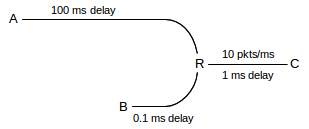In the diagram above, the A–C connection wants its cwnd to be about 200 ms × 10 packets/ms = 2,000 packets; it is competing for the R–C link with the B–D connection which is happy with a cwnd of 22. If R’s queue capacity is also about 20, then with most of the bandwidth the B–C connection will experience a loss about every 20 RTTs, which is to say every 22 ms. If the A–C link shares even a modest fraction of those losses, it is indeed in trouble.

However, the A–C cwnd cannot fall below 1.0; to test the 10,000-fold hypothesis taking this constraint into account we would have to scale up the numbers on the B–C link so the transit capacity there was at least 10,000. This would mean a 400 Gbps R–C bandwidth, or else an unrealistically large A–R delay.

As a second issue, realistically the A–C link is much more likely to have its bottleneck somewhere in the middle of its long path. In a typical real scenario along the lines of that diagrammed above, B, C and R are all local to a site, and bandwidth of long-haul paths is almost always less than the local LAN bandwidth within a site. If the A–R path has a 1 packet/ms bottleneck somewhere, then it may be less likely to be as dramatically affected by B–C traffic.

A few actual simulations using the methods of 16.3   Two TCP Senders Competing resulted in an average cwnd for the A–C connection of between 1 and 2, versus a B–C cwnd of 20-25, regardless of whether the two links shared a bottleneck or if the A–C link had its bottleneck somewhere along the A–R path. This may suggest that the A–C connection was indeed saved by the 1.0 cwnd minimum.

## 14.4   Notions of Fairness¶

There are several definitions for fair allocation of bandwidth among flows sharing a bottleneck link. One is equal-shares fairness; another is what we might call TCP-Reno fairness: to divide the bandwidth the way TCP Reno would. There are additional approaches to deciding what constitutes a fair allocation of bandwidth.

### 14.4.1   Max-Min Fairness¶

A natural generalization of equal-shares fairness to the case where some flows may be capped is max-min fairness, in which no flow bandwidth can be increased without decreasing some smaller flow rate. Alternatively, we maximize the bandwidth of the smallest-capacity flow, and then, with that flow fixed, maximize the flow with the next-smallest bandwidth, etc. A more intuitive explanation is that we distribute bandwidth in tiny increments equally among the flows, until the bandwidth is exhausted (meaning we have divided it equally), or one flow reaches its externally imposed bandwidth cap. At this point we continue incrementing among the remaining flows; any time we encounter a flow’s external cap we are done with it.

As an example, consider the following, where we have connections A–D, B–D and C–D, and where the A–R link has a bandwidth of 200 Kbps and all other links are 1000 Kbps. Starting from zero, we increment the allocations of each of the three connections until we get to 200 Kbps per connection, at which point the A–D connection has maxed out the capacity of the A–R link. We then continue allocating the remaining 400 Kbps equally between B–D and C–D, so they each end up with 400 Kbps.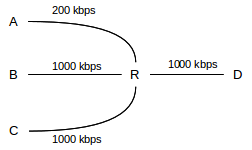As another example, known as the parking-lot topology, suppose we have the following network: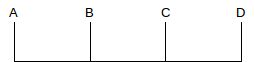There are four connections: one from A to D covering all three links, and three single-link connections A–B, B–C and C–D. Each link has the same bandwidth. If bandwidth allocations are incrementally distributed among the four connections, then the first point at which any link bandwidth is maxed out occurs when all four connections each have 50% of the link bandwidth; max-min fairness here means that each connection has an equal share.

### 14.4.2   Proportional Fairness¶

A bandwidth allocation of rates ⟨r1,r2,...,rN⟩ for N connections satisfies proportional fairness if it is a legal allocation of bandwidth, and for any other allocation ⟨s1,s2,...,sN⟩, the aggregate proportional change satisfies

(r1−s1)/s1 + (r2−s2)/s2 + ... + (rN−sN)/sN < 0

Alternatively, proportional fairness means that the sum log(r1)+log(r2)+...+log(rN) is minimized. If the connections share only the bottleneck link, proportional fairness is achieved with equal shares. However, consider the following two-stage parking-lot network: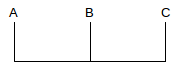Suppose the A–B and B–C links have bandwidth 1 unit, and we have three connections A–B, B–C and A–C. Then a proportionally fair solution is to give the A–C link a bandwidth of 1/3 and each of the A–B and B–C links a bandwidth of 2/3 (so each link has a total bandwidth of 1). For any change 𝚫b in the bandwidth for the A–C link, the A–B and B–C links each change by -𝚫b. Equilibrium is achieved at the point where a 1% reduction in the A–C link results in two 0.5% increases, that is, the bandwidths are divided in proportion 1:2. Mathematically, if x is the throughput of the A–C connection, we are minimizing log(x) + 2log(1-x).

Proportional fairness partially addresses the problem of TCP Reno’s bias against long-RTT connections; specifically, TCP’s bias here is still not proportionally fair, but TCP’s response is closer to proportional fairness than it is to max-min fairness.

## 14.5   TCP Reno loss rate versus cwnd¶

It turns out that we can express a connection’s average cwnd in terms of the packet loss rate, p, eg p = 10-4 = one packet lost in 10,000. The relationship comes by assuming that all packet losses are because the network ceiling was reached. We will also assume that, when the network ceiling is reached, only one packet is lost, although we can dispense with this by counting a “cluster” of related losses (within, say, one RTT) as a single loss event.

Let C represent the network ceiling – so that when cwnd reaches C a packet loss occurs. While the assumption that C is constant represents a very stable network, the general case is not terribly different. Then cwnd varies between C/2 and C, with packet drops occurring whenever cwnd = C is reached. Let N = C/2. Then between two consecutive packet loss events, that is, over one “tooth” of the TCP connection, a total of N+(N+1)+ ... +2N packets are sent in N+1 flights; this sum can be expressed algebraically as 3/2 N(N+1) ≃ 1.5 N2. The loss rate is thus one packet out of every 1.5 N2, and the loss rate p is 1/(1.5 N2).

The average cwnd in this scenario is 3/2 N (that is, the average of N=cwndmin and 2N=cwndmax). If we let M = 3/2 N represent the average cwnd, cwndmean, we can express the above loss rate in terms of M: the number of packets between losses is 2/3 M2, and so p=3/2 M-2.

Now let us solve this for M=cwndmean in terms of p; we get M2 = 3/2 p-1 and thus

M = cwndmean = 1.225 p-1/2

where 1.225 is the square root of 3/2. Seen in this form, a given network loss rate sets the window size; this loss rate is ultimately be tied to the network capacity. If we are interested in the maximum cwnd instead of the mean, we multiply the above by 4/3.

From the above, the bandwidth available to a connection is now as follows (though RTT may not be constant):

bandwidth = cwnd/RTT = 1.225/(RTT × √p)

In [PFTK98] the authors consider a TCP Reno model that takes into account the measured frequency of coarse timeouts (in addition to fast-recovery responses leading to cwnd halving), and develop a related formula with additional terms.

### 14.5.1   Irregular teeth¶

In the preceding, we assumed that all teeth were the same size. What if they are not? In [OKM96], this problem was considered under the assumption that every packet faces the same (small) loss probability (and so the intervals between packet losses are exponentially distributed). In this model, it turns out that the above formula still holds except the constant changes from 1.225 to 1.309833.

To understand how irregular teeth lead to a bigger constant, imagine sending a large number K of packets which encounter n losses. If the losses are regularly spaced, then the TCP graph will have n equally sized teeth, each with K/n packets. But if the n losses are randomly distributed, some teeth will be larger and some will be smaller. The average tooth height will be the same as in the regularly-spaced case (see exercise 13). However, the number of packets in any one tooth is generally related to the square of the height of that tooth, and so larger teeth will count disproportionately more. Thus, the random distribution will have a higher total number of packets delivered and thus a higher mean cwnd.

See also exercise 17, for a simple simulation that generates a numeric estimate for the constant 1.309833.

Note that losses at uniformly distributed random intervals may not be an ideal model for TCP either; in the presence of congestion, loss events are far from statistical independence. In particular, immediately following one loss another loss is unlikely to occur until the queue has time to fill up.

### 14.5.2   Unsynchronized TCP Losses¶

In 14.3.3   TCP RTT bias we considered a model in which all loss events are fully synchronized; that is, whenever the queue becomes full, both TCP Reno connections always experience packet loss. In that model, if RTT2/RTT1 = 𝜆 then cwnd1/cwnd2 = 𝜆 and bandwidth1/bandwidth2 = 𝜆2, where cwnd1 and cwnd2 are the respective average values for cwnd.

What happens if loss events for two connections do not have such a neat one-to-one correspondence? We will derive the ratio of loss events (or, more precisely, TCP loss responses) for connection 1 versus connection 2 in terms of the bandwidth and RTT ratios, without using the synchronized-loss hypothesis.

Note that we are comparing the total number of loss events (or loss responses) here – the total number of TCP Reno teeth – over a large time interval, and not the relative per-packet loss probabilities. One connection might have numerically more losses than a second connection but, by dint of a smaller RTT, send more packets between its losses than the other connection and thus have fewer losses per packet.

Let losscount1 and losscount2 be the number of loss responses for each connection over a long time interval T. The ith connection’s per-packet loss probability is pi = losscounti/(bandwidthi × T) = (losscounti × RTTi)/(cwndi× T). But by the result of 14.5   TCP Reno loss rate versus cwnd, we also have cwndi = k/√pi, or pi = k2/cwndi2. Equating, we get

pi = k2/cwndi2 = (losscounti × RTTi) / (cwndi × T)

and so

losscounti = k2T / (cwndi × RTTi)

Dividing and canceling, we get

losscount1/losscount2 = (cwnd2/cwnd1) × (RTT2/RTT1)

We will make use of this in 16.4.2.2   Relative loss rates.

We can go just a little further with this: let 𝛾 denote the losscount ratio above:

𝛾 = (cwnd2/cwnd1) × (RTT2/RTT1)

Therefore, as RTT2/RTT1 = 𝜆, we must have cwnd2/cwnd1 = 𝛾/𝜆 and thus

bandwidth1/bandwidth2 = (cwnd1/cwnd2) × (RTT2/RTT1) = 𝜆2/𝛾.

Note that if 𝛾=𝜆, that is, if the longer-RTT connection has fewer loss events in exact inverse proportion to the RTT, then bandwidth1/bandwidth2 = 𝜆 = RTT2/RTT1, and also cwnd1/cwnd2 = 1.

## 14.6   TCP Friendliness¶

Suppose we are sending packets using a non-TCP real-time protocol. How are we to manage congestion? In particular, how are we to manage congestion in a way that treats other connections – particularly TCP Reno connections – fairly?

For example, suppose we are sending interactive audio data in a congested environment. Because of the real-time nature of the data, we cannot wait for lost-packet recovery, and so must use UDP rather than TCP. We might further suppose that we can modify the encoding so as to reduce the sending rate as necessary – that is, that we are using adaptive encoding – but that we would prefer in the absence of congestion to keep the sending rate at the high end. We might also want a relatively uniform rate of sending; the TCP sawtooth leads to periodic variations in throughput that we may wish to avoid.

Our application may not be windows-based, but we can still monitor the number of packets it has in flight on the network at any one time; if the packets are small, we can count bytes instead. We can use this count instead of the TCP cwnd.

We will say that a given communications strategy is TCP Friendly if the number of packets on the network at any one time is approximately equal to the TCP Reno cwndmean for the prevailing packet loss rate p. Note that – assuming losses are independent events, which is definitely not quite right but which is often Close Enough – in a long-enough time interval, all connections sharing a common bottleneck can be expected to experience approximately the same packet loss rate.

The point of TCP Friendliness is to regulate the number of the non-Reno connection’s outstanding packets in the presence of competition with TCP Reno, so as to achieve a degree of fairness. In the absence of competition, the number of any connection’s outstanding packets will be bounded by the transit capacity plus capacity of the bottleneck queue. Some non-Reno protocols (eg TCP Vegas, 15.4   TCP Vegas, or constant-rate traffic, 14.6.2   RTP) may in the absence of competition have a loss rate of zero, simply because they never overflow the queue.

Another way to approach TCP Friendliness is to start by defining “Reno Fairness” to be the bandwidth allocations that TCP Reno assigns in the face of competition. TCP Friendliness then simply means that the given non-Reno connection will get its Reno-Fair share – not more, not less.

We will return to TCP Friendliness in the context of general AIMD in 14.7   AIMD Revisited.

### 14.6.1   TFRC¶

TFRC, or TCP-Friendly Rate Control, RFC 3448, uses the loss rate experienced, p, and the formulas above to calculate a sending rate. It then allows sending at that rate; that is, TFRC is rate-based rather than window-based. As the loss rate increases, the sending rate is adjusted downwards, and so on. However, adjustments are done more smoothly than with TCP.

From RFC 5348:

TFRC is designed to be reasonably fair when competing for bandwidth with TCP flows, where we call a flow “reasonably fair” if its sending rate is generally within a factor of two of the sending rate of a TCP flow under the same conditions. [emphasis added; a factor of two might not be considered “close enough” in some cases.]

The penalty of having smoother throughput than TCP while competing fairly for bandwidth is that TFRC responds more slowly than TCP to changes in available bandwidth.

TFRC senders include in each packet a sequence number, a timestamp, and an estimated RTT.

The TFRC receiver is charged with sending back feedback packets, which serve as (partial) acknowledgments, and also include a receiver-calculated value for the loss rate over the previous RTT. The response packets also include information on the current actual RTT, which the sender can use to update its estimated RTT. The TFRC receiver might send back only one such packet per RTT.

The actual response protocol has several parts, but if the loss rate increases, then the primary feedback mechanism is to calculate a new (lower) sending rate, using some variant of the cwnd = k/√p formula, and then shift to that new rate. The rate would be cut in half only if the loss rate p quadrupled.

Newer versions of TFRC have a various features for responding more promptly to an unusually sudden problem, but in normal use the calculated sending rate is used most of the time.

### 14.6.2   RTP¶

The Real-Time Protocol, or RTP, is sometimes (though not always) coupled with TFRC. RTP is a UDP-based protocol for streaming time-sensitive data.

Some RTP features include:

• The sender establishes a rate (rather than a window size) for sending packets
• The receiver returns periodic summaries of loss rates
• ACKs are relatively infrequent
• RTP is suitable for multicast use; a very limited ACK rate is important when every packet sent might have hundreds of recipients
• The sender adjusts its cwnd-equivalent up or down based on the loss rate and the TCP-friendly cwnd=k/√p rule
• Usually some sort of “stability” rule is incorporated to avoid sudden changes in rate

As a common RTP example, a typical VoIP connection using a DS0 (64 Kbps) rate might send one packet every 20 ms, containing 160 bytes of voice data, plus headers.

For a combination of RTP and TFRC to be useful, the underlying application must be rate-adaptive, so that the application can still function when the available rate is reduced. This is often not the case for simple VoIP encodings; see 18.11.4   RTP and VoIP.

We will return to RTP in 18.11   Real-time Transport Protocol (RTP).

## 14.7   AIMD Revisited¶

TCP Tahoe chose an increase increment of 1 on no losses, and a decrease factor of 1/2 otherwise.

Another approach to TCP Friendliness is to retain TCP’s additive-increase, multiplicative-decrease strategy, but to change the numbers. Suppose we denote by AIMD(𝛼,𝛽) the strategy of incrementing the window size by 𝛼 after a window of no losses, and multiplying the window size by (1-𝛽)<1 on loss (so 𝛽=0.1 means the window is reduced by 10%). TCP Reno is thus AIMD(1,0.5).

Any AIMD(𝛼,𝛽) protocol also follows a sawtooth, where the slanted top to the tooth has slope 𝛼. All combinations of 𝛼>0 and 0<𝛽<1 are possible. The dimensions of one tooth of the sawtooth are somewhat constrained by 𝛼 and 𝛽. Let h be the maximum height of the tooth and let w be the width (as measured in RTTs). Then, if the losses occur at regular intervals, the height of the tooth at the left (low) edge is (1-𝛽)h and the total vertical difference is 𝛽h. This vertical difference must also be 𝛼w, and so we get 𝛼w = 𝛽h, or h/w = 𝛼/𝛽; these values are labeled on the rightmost teeth in the diagram below. These equations mean that the proportions of the tooth (h to w) are determined by 𝛼 and 𝛽. Finally, the mean height of the tooth is (1-𝛽/2)h.

We are primarily interested in AIMD(𝛼,𝛽) cases which are TCP Friendly (14.6   TCP Friendliness). TCP friendliness means that an AIMD(𝛼,𝛽) connection with the same loss rate as TCP Reno will have the same mean cwnd. Each tooth of the sawtooth represents one loss. The number of packets sent per tooth is, using h and w as in the previous paragraph, (1-𝛽/2)hw.

Geometrically, the number of packets sent per tooth is the area of the tooth, so two connections with the same per-packet loss rate will have teeth with the same area. TCP Friendliness means that two connections will have the same mean cwnd and thus the same tooth height. If the teeth of two connections have the same area and the same height, they must have the same width (in RTTs), and thus that the rates of loss per unit time must be equal, not just the rates of loss per number of packets.

The diagram below shows a TCP Reno tooth (blue) together with some unfriendly AIMD(𝛼,𝛽) teeth on the left (red) and two friendly teeth on the right (green), the second friendly tooth is superimposed on the Reno tooth.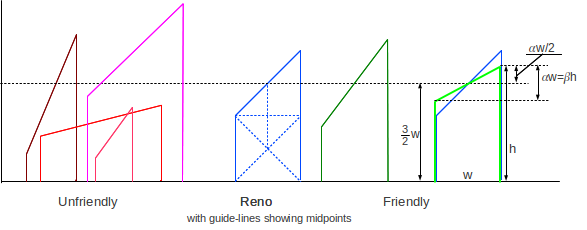The additional dashed lines within the central Reno tooth demonstrate the Reno 1×1×2 dimensions, and show that the horizontal dashed line, representing cwndmean, is at height 3/2 w, where w is, as before, the width.

In the rightmost green tooth, superimposed on the Reno tooth, we can see that h = (3/2)×w + (𝛼/2)×w. We already know h = (𝛼/𝛽)×w; setting these expressions equal, canceling the w and multiplying by 2 we get (3+𝛼) = 2𝛼/𝛽, or 𝛽 = 2𝛼/(3+𝛼). Solving for 𝛽 we get

𝛼 = 3𝛽/(2-𝛽)

or 𝛼 ≃ 1.5𝛽 for small 𝛽. As the reduction factor 1-𝛽 gets closer to 1, the protocol can remain TCP-friendly by appropriately reducing 𝛼; eg AIMD(1/5, 1/8).

Having a small 𝛽 means that a connection does not have sudden bandwidth drops when losses occur; this can be important for applications that rely on a regular rate of data transfer (such as voice). Such applications are sometimes said to be slowly responsive, in contrast to TCP’s cwnd = cwnd/2 rapid response.

### 14.7.1   AIMD and Convergence to Fairness¶

While TCP-friendly AIMD(𝛼,𝛽) protocols will converge to fairness when competing with TCP Reno (with equal RTTs), a consequence of decreasing 𝛽 is that fairness may take longer to arrive; here is an example. We will assume, as above in 14.3.3   TCP RTT bias, that loss events for the two competing connections are synchronized. Recall that for two same-RTT TCP Reno connections (that is, AIMD(𝛼,𝛽) where 𝛽=1/2), if the initial difference in the connections’ respective cwnds is D, then D is reduced by half on each loss event.

Now suppose we have two AIMD(𝛼,𝛽) connections with some other value of 𝛽, and again with a difference D in their cwnd values. The two connections will each increase cwnd by 𝛼 each RTT, and so when losses are not occurring D will remain constant. At loss events, D will be reduced by a factor of 1-𝛽. If 𝛽=1/4, corresponding to 𝛼=3/7, then at each loss event D will be reduced only to 3/4 D, and the “half-life” of D will be almost twice as large. The two connections will still converge to fairness as D→0, but it will take twice as long.

## 14.8   Active Queue Management¶

Active Queue Management means that routers take some active steps to manage their queues. Generally this means either marking packets or dropping them. All routers drop packets, but this falls into the category of active management when packets are dropped before the queue has run completely out of space. Queue management can be done at the congestion “knee”, when queues just start to build (and when marking is more appropriate), or as the queue starts to become full and approaches the “cliff”.

### 14.8.1   DECbit¶

In the congestion-avoidance technique proposed in [RJ90], routers encountering early signs of congestion marked the packets they forwarded; senders used these markings to adjust their window size. The system became known as DECbit in reference to the authors’ employer and was implemented in DECnet (closely related to the OSI protocol suite), though apparently there was never a TCP/IP implementation. The idea behind DECbit eventually made it into TCP/IP in the form of ECN, below, but while ECN – like TCP’s other congestion responses – applies control near the congestion cliff, DECbit proposed introducing control when congestion was still minimal, just above the congestion knee.

The DECbit mechanism allowed routers to set a designated “congestion bit”. This would be set in the data packet being forwarded, but the status of this bit would be echoed back in the corresponding ACK (otherwise the sender would never hear about the congestion).

DECbit routers defined “congestion” as an average queue size greater than 1.0; that is, congestion meant that the connection was just past the “knee”. Routers would set the congestion bit whenever this average-queue condition was met.

The target for DECbit senders would then be to have 50% of packets marked as “congested”. If fewer than 50% of packets were marked, cwnd would be incremented by 1; if more than 50% were marked, then cwnd would be decreased by a factor of 0.875. Note this is very different from the TCP approach in that DECbit begins marking packets at the congestion “knee” while TCP Reno responds only to packet losses which occur just over the “cliff”.

A consequence of this knee-based mechanism is that DECbit shoots for very limited queue utilization, unlike TCP Reno. At a congested router, a DECbit connection would attempt to keep about 1.0 packets in the router’s queue, while a TCP Reno connection might fill the remainder of the queue. Thus, DECbit would in principle compete poorly with any connection where the sender ignored the marked packets and simply tried to keep cwnd as large as possible. As we will see in 15.4   TCP Vegas, TCP Vegas also strives for limited queue utilization; in 16.5   TCP Reno versus TCP Vegas we investigate through simulation how fairly TCP Vegas competes with TCP Reno.

### 14.8.2   Explicit Congestion Notification (ECN)¶

ECN is the TCP/IP equivalent of DECbit, though the actual mechanics are quite different. The current version is specified in RFC 3168, modifying an earlier version in RFC 2481. The IP header contains a two-bit ECN field, consisting of the ECN-Capable Transport (ECT) bit and the Congestion Experienced (CE) bit; these are shown in 7.1   The IPv4 Header. The ECT bit is set by a sender to indicate to routers that it is able to use the ECN mechanism. (These are actually the older RFC 2481 names for the bits, but they will serve our purposes here.) The TCP header contains an additional two bits: the ECN-Echo bit (ECE) and the Congestion Window Reduced (CWR) bit; these are shown in the fourth row in 12.2   TCP Header.

Routers set the CE bit in the IP header when they might otherwise drop the packet (or possibly when the queue is at least half full, or in lieu of a RED drop, below). As in DECbit, receivers echo the CE status back to the sender in the ECE bit of the next ACK; the reason for using the ECE bit is that this bit belongs to the TCP header and thus the TCP layer can be assured of control of it.

TCP senders treat ACKs with the ECE bit set the same as if a loss occurred: cwnd is cut in half. Because there is no actual loss, the arriving ACKs can still pace continued sliding-windows sending. The Fast Recovery mechanism is not needed.

When the TCP sender has responded to an ECE bit, it sets the CWR bit. Once the receiver has received a packet with the CE bit set in the IP layer, it sets the ECE bit in all subsequent ACKs until it receives a data packet with the CWR bit set. This provides for reliable communication of the congestion information, and helps the sender respond just once to multiple packet losses within a single windowful.

Note that the initial packet marking is done at the IP layer, but the generation of the marked ACK and the sender response to marked packets is at the TCP layer (the same is true of DECbit though the layers have different names).

Another advantage of the ECN mechanism, in addition to avoiding actual packet loss, is that the sender will discover the congestion within a single RTTnoLoad, rather than waiting for the existing queue to be transmitted. If a router’s queue reaches the router’s congestion-notification threshold, the very next packet forwarded (at the head of the queue) can have the ECN bit set. If a packet is dropped, however, the drop occurs upon arrival at the bottleneck router but is not discovered by the sender until every packet in the queue has been delivered and acknowledged, and the sender is in a position to notice the missing ACK. By then, other losses may have occurred.

Even with ECN, however, the marked packet must still reach its destination and be acknowledged before the sender finds out about the marking. A much earlier, “legacy” strategy was to require routers, upon dropping a packet, to immediately send back to the sender an ICMP Source Quench packet. This is the fastest possible way to notify a sender of a loss. It was never widely implemented, however, and was officially deprecated by RFC 6633.

Because ECN congestion is treated the same way as packet drops, ECN competes fairly with TCP Reno; the slightly earlier notification about packet loss does not materially affect bandwidth allocation.

### 14.8.3   RED gateways¶

“Standard” routers drop packets only when the queue is full; senders have no overt indication before then that the cliff is looming. The idea behind Random Early Detection (RED) gateways, introduced in [FJ93], is that the router is allowed to drop an occasional packet much earlier, say when the queue is only half full. These early packet drops provide a signal to senders that they should slow down; we will call them signaling losses. While packets are indeed lost, they are dropped in such a manner that usually only one packet per windowful (per connection) will be lost. Classic TCP Reno, in particular, behaves poorly with multiple losses per window and RED is able to avoid such multiple losses.

RED is, in a technical sense, its own “queuing discipline”; we address these further in 17   Queuing and Scheduling. However, it is often more helpful to think of RED as a technique that an otherwise-FIFO router can use to improve the performance of TCP traffic through it.

Tuning RED has taken some thought. An earlier version known as Early Random Drop (ERD) gateways simply introduced a small uniform drop probability p, eg p=0.01, once the queue had reached a certain threshold. This addresses the TCP Reno issue reasonably well, except that dropping with a uniform probability p leads to a surprisingly high rate of multiple drops in a cluster, or of long stretches with no drops. More uniformity was needed, but drops at regular intervals are too uniform.

The actual RED algorithm does two things. First, the base drop probability – pbase – rises steadily from a minimum queue threshold qmin to a maximum queue threshold qmax (these might be 40% and 80% respectively of the absolute queue capacity); at the maximum threshold, the drop probability is still quite small. The base probability pbase increases linearly in this range according to the following formula, where pmax is the maximum RED-drop probability; the value for pmax proposed in [FJ93] was 0.02.

pbase = pmax × (avg_queuesize − qmin)/(qmax − qmin)

Second, as time passes after a RED drop, the actual drop probability pactual begins to rise, according to the next formula:

pactual = pbase / (1 − count×pbase)

Here, count is the number of packets sent since the last RED drop. With count=0 we have pactual = pbase, but pactual rises from then on with a RED drop guaranteed within the next 1/pbase packets. This provides a mechanism by which RED drops are uniformly enough spaced that it is unlikely two will occur in the same window of the same connection, and yet random enough that it is unlikely that the RED drops will remain synchronized with a single connection, thus targeting it unfairly.

One potential RED drawback is that the choice of the various parameters is decidedly ad hoc, and it is not clear how to set them so that TCP connections with both small and large bandwidth×delay products are handled appropriately. The probability pbase should, for example, be roughly 1/winsize, but winsize for TCP connections can vary by several orders of magnitude. Nonetheless, RED gateways have worked quite well in practice.

In 18.8   RED with In and Out we will look at an application of RED to quality-of-service guarantees.

## 14.9   The High-Bandwidth TCP Problem¶

The TCP Reno algorithm has a serious consequence for high-bandwidth connections: the cwnd needed implies a very small – unrealistically small – packet-loss rate p. “Noise” losses (losses not due to congestion) are not frequent but no longer negligible; these keep the window significantly smaller than it should be. The following table is based on an RTT of 0.1 seconds and a packet size of 1500 bytes, for various throughputs. The cwnd values represent the bandwidth×RTT products.

TCP Throughput (Mbps) RTTs between losses cwnd Packet Loss Rate P
1 5.5 8.3 0.02
10 55 83 0.0002
100 555 833 0.000002
1000 5555 8333 0.00000002
10,000 55555 83333 2 × 10-10

Note the very small loss probability needed to support 10 Gbps; this works out to a bit error rate of less than 2 × 10-14. For fiber optic data links, alas, a physical bit error rate of 10-13 is often considered acceptable; there is thus no way to support the window size of the final row above.

Here is a similar table, expressing cwnd in terms of the packet loss rate:

Packet Loss Rate P cwnd RTTs between losses
10-2 12 8
10-3 38 25
10-4 120 80
10-5 379 252
10-6 1,200 800
10-7 3,795 2,530
10-8 12,000 8,000
10-9 37,948 25,298
10-10 120,000 80,000

The above two tables indicate that large window sizes require extremely small drop rates. This is the high-bandwidth-TCP problem: how do we maintain a large window when a path has a large bandwidth×delay product? The primary issue is that non-congestive (noise) packet losses bring the window size down, potentially far below where it could be. A secondary issue is that, even if such random drops are not significant, the increase of cwnd to a reasonable level can be quite slow. If the network ceiling were about 2,000 packets, then the normal sawtooth return to the ceiling after a loss would take 1,000 RTTs. This is slow, but the sender would still average 75% throughput, as we saw in 13.7   TCP and Bottleneck Link Utilization. Perhaps more seriously, if the network ceiling were to double to 4,000 packets due to decreases in competing traffic, it would take the sender an additional 2,000 RTTs to reach the point where the link was saturated.

In the following diagram, the network ceiling and the ideal TCP sawtooth are shown in green. The ideal TCP sawtooth should range between 50% and 100% of the ceiling; in the diagram, “noise” or non-congestive losses occur at the red x’s, bringing down the throughput to a much lower average level.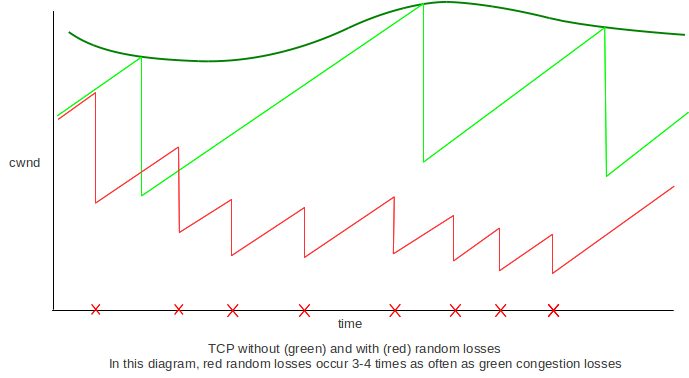## 14.12   Epilog¶

TCP Reno’s core congestion algorithm is based on algorithms in Jacobson and Karel’s 1988 paper [JK88], now twenty-five years old. There are concerns both that TCP Reno uses too much bandwidth (the greediness issue) and that it does not use enough (the high-bandwidth-TCP problem).

In the next chapter we consider alternative versions of TCP that attempt to solve some of the above problems associated with TCP Reno.

## 14.13   Exercises¶

1. Consider the following network, where the bandwidths marked are all in packets/ms. C is sending to D using sliding windows and A and B are idle.

          C
│
100
│
A───100───R1───5───R2───100───B
│
100
│
D

Suppose the propagation delay on the 100 packet/ms links is 1 ms, and the propagation delay on the R1–R2 link is 2 ms. The RTTnoLoad for the C–D path is thus about 8 ms, for a bandwidth×delay product of 40 packets. If C uses winsize = 50, then the queue at R1 will have size 10.

Now suppose A starts sending to B using sliding windows, also with winsize = 50. What will be the size of the queue at R1?

Hint: by symmetry, the queue will be equally divided between A’s packets and C’s, and A and C will each see a throughput of 2.5 packets/ms. RTTnoLoad, however, does not change.

2. In the previous exercise, give the average number of data packets in transit on each link:

(a). for the original case in which C is the only sender, with winsize = 50 (the only active links here are C–R1, R1–R2 and R2–D).
(b). for the new case in which B is also sending, also with winsize = 50. In this case all links are active.

Each link will also have an equal number of ACK packets in transit in the reverse direction.

3. Consider the C–D path from the diagram of 14.2.4   Example 4: cross traffic and RTT variation:

C───100───R1───5───R2───100───D

Link numbers are bandwidths in packets/ms. Assume C is the only sender.

(a). Give propagation delays for the links C–R1 and R2–D so that there will be an average of 5 packets in transit on the C–R1 and R2–D links, in each direction, if C uses a winsize sufficient to saturate the bottleneck R1–R2 link.
(b). Give propagation delays for all three links so that, when C uses a winsize equal to the round-trip transit capacity, there are 5 packets each way on the C–R1 link, 10 on the R1–R2 link, and 20 on the R2–D link.

4. Suppose we have the network layout below of 14.2.4   Example 4: cross traffic and RTT variation, except that the R1–R2 bandwidth is 6 packets/ms and the R2–R3 bandwidth is 3. Suppose also that A and C have settled upon window sizes so that each contributes 30 packets to R1’s queue – and thus each has 50% of the bandwidth. R2 will then be sending 3 packets/ms to R3 and so will have no queue.

Now A’s winsize is incremented by 10, initially, at least, leading to A contributing more than 50% of R1’s queue. When the steady state is reached, how will these extra 10 packets be distributed between R1 and R2? Hint: As A’s winsize increases, A’s overall throughput cannot rise due to the bandwidth restriction of the R2–R3 link.

          C
│
100
│
A───100───R1───6───R2───3───R3───100───B
│
100
│
D

Diagram for exercises 4 and 5

5. Suppose we have the network layout above similar to 14.2.4   Example 4: cross traffic and RTT variation for which we are given that the round-trip C–D RTTnoLoad is 5 ms. The round-trip A–B RTTnoLoad may be different.

The R1–R2 bandwidth is 6 packets/ms, so with A idle the C–D The R2–R3 bandwidth is 3 packets/ms.

(a). Suppose that A and C have window sizes such that, with both transmitting, each has 30 packets in the queue at R1. What is C’s winsize? Hint: C’s bandwidth is now 3 packets/ms.
(b). Now suppose C’s winsize, with A idle, is 60. In this case the C–D transit capacity would be 5 ms × 6 packets/ms = 30 packets, and so C would have 60−30 = 30 packets in R1’s queue. A then begins sending, with a winsize chosen so that A and C’s contributions to R1’s queue are equal; C’s winsize remains at 60. What will be C’s (and thus A’s) queue usage at R1? Hint: find the transit capacity for a bandwidth of 3 packets/ms.
(c). Suppose the A–B RTTnoLoad is 10 ms. If C’s winsize is 60, find the winsize for A that makes A and C’s contributions to R1’s queue equal.

6. One way to address the reduced bandwidth TCP Reno gives to long-RTT connections is for all connections to use an increase increment of RTT2 instead of 1; that is, everyone uses AIMD(RTT2,1/2) instead of AIMD(1,1/2) (or AIMD(k×RTT2,1/2), where k is an arbitrary scaling factor that applies to everyone).

(a). Construct a table in the style of of 14.3.2   Example 3: Longer RTT above, showing the result of two connections using this strategy, where one connection has RTT = 1 and the other has RTT = 2. Start the connections with cwnd=RTT2, and assume a loss occurs when cwnd1 + cwnd2 > 24.
(b). Explain why this strategy might not be desirable if one connection is over a direct LAN with an RTT of 1 ms, while the second connection has a very long path and an RTT of 1.0 sec.

7. For each value 𝛼 or 𝛽 below, find the other value so that AIMD(𝛼,𝛽) is TCP-friendly.

(a). 𝛽 = 1/5
(b). 𝛽 = 2/9
(c). 𝛼 = 1/5

Then pick the pair that has the smallest 𝛼, and draw a sawtooth diagram that is approximately proportional: 𝛼 should be the slope of the linear increase, and 𝛽 should be the decrease fraction at the end of each tooth.

8. Suppose two TCP flows compete. The first flow uses AIMD(𝛼1,𝛽1) and the second uses AIMD(𝛼2,𝛽2). The two connections compete fairly; that is, they have the same average packet-loss rates. Show that 𝛼1/𝛽1 = (2-𝛽2)/(2-𝛽1) × 𝛼2/𝛽2. Assume regular losses, and use the methods of 14.7   AIMD Revisited.

9. Suppose two 1K packets are sent as part of a packet-pair probe, and the minimum time measured between arrivals is 5 ms. What is the estimated bottleneck bandwidth?

10. Consider the following three causes of a 1-second network delay between A and B. In all cases, assume ACKs travel instantly from B back to A.

(i) An intermediate router with a 1-second-per-packet bandwidth delay; all other bandwidth delays negligible
(ii) An intermediate link with a 1-second propagation delay; all bandwidth delays negligible
(iii) An intermediate router with a 100-ms-per-packet bandwidth delay, and a steadily replenished queue of 10 packets, from another source (as in the diagram in 14.2.4   Example 4: cross traffic and RTT variation).

How might a sender distinguish between these three cases? Assume that the sender is willing to create special “probe” packet arrangements.

11. Consider again the three-link parking-lot network from 14.4.1   Max-Min Fairness:(a). Suppose we have two end-to-end connections, in addition to one single-link connection for each link. Find the max-min-fair allocation.
(b). Suppose we have a single end-to-end connection, and one B–C and C–D connection, but two A–B connections. Find the max-min-fair allocation.

12. Consider the two-link parking-lot network:

A       B       C
│       │       │
└───────┴───────┘

Suppose there are two A–C connections, one A–B connection and one A–C connection. Find the allocation that is proportionally fair.

13. Suppose we use TCP Reno to send K packets over R RTT intervals. The transmission experiences n not-necessarily-uniform loss events; the TCP cwnd graph thus has n sawtooth peaks of heights N1 through Nn. At the start of the graph, cwnd = A, and at the end of the graph, cwnd = B. Show that the sum N1 + ... + Nn is 2(R+A-B), and in particular the average tooth height is independent of the distribution of the loss events.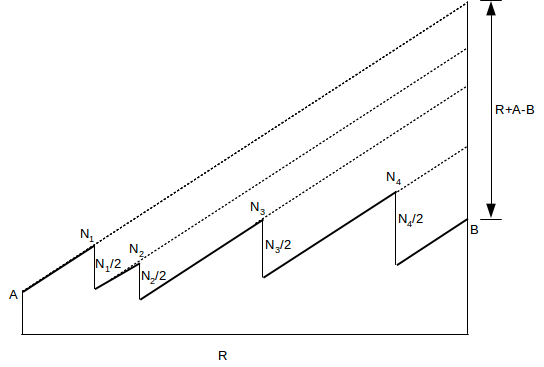14. Suppose TCP Reno has regularly spaced sawtooth peaks of the same height, but the packet losses come in pairs, with just enough separation that both losses in a pair are counted separately. N is large enough that the spacing between the two losses is negligible. The net effect is that each large-scale tooth ranges from height N/4 to N. As in 14.5   TCP Reno loss rate versus cwnd, the ratio of the average cwnd to the square root of the loss rate p, cwnd/√p, is constant. Find the constant. Note that the loss rate here is p = 2/(number of packets sent in one tooth).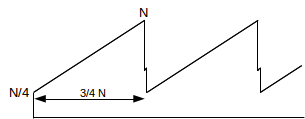15. As in the previous exercise, suppose a TCP transmission has large-scale teeth of height N. Between each pair of consecutive large teeth, however, there are K-1 additional losses resulting in K-1 additional tiny teeth; N is large enough that these tiny teeth can be ignored. A non-Reno variant of TCP is used, so that between these tiny teeth cwnd is assumed not to be cut in half; during the course of these tiny teeth cwnd does not change much at all. The large-scale tooth has width N/2 and height ranging from N/2 to N, and there are K losses per large-scale tooth. Find the ratio cwnd/√p, in terms of K. When K=1 your answer should reduce to that derived in 14.5   TCP Reno loss rate versus cwnd.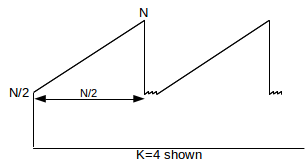16. Suppose a TCP Reno tooth starts with cwnd = c, and contains N packets. Let w be the width of the tooth, in RTTs as usual. Show that w = (c2 + 2N)1/2 − c. Hint: the maximum height of the tooth will be c+w, and so the average height will be c + w/2. Find an equation relating c, w and N, and solve for w using the quadratic formula.

17. Suppose in a TCP Reno run each packet is equally likely to be lost; the number of packets N in each tooth will therefore be distributed exponentially. That is, N = -k log(X), where X is a uniformly distributed random number in the range 0<X<1 (k, which does not really matter here, is the mean interval between losses). Write a simple program that simulates such a TCP Reno run. At the end of the simulation, output an estimate of the constant C in the formula cwndmean = C/√p. You should get a value of about 1.31, as in the formula in 14.5.1   Irregular teeth.

Hint: There is no need to simulate packet transmissions; we simply create a series of teeth of random size, and maintain running totals of the number of packets sent, the number of RTT intervals needed to send them, and the number of loss events (that is, teeth). After each loss event (each tooth), we update:

• total_packets += packets sent in this tooth
• RTT_intervals += RTT intervals in this tooth
• loss_events += 1 (one tooth = one loss event)

If a loss event marking the end of one tooth occurs at a specific value of cwnd, the next tooth begins at height c = cwnd/2. If N is the random value for the number of packets in this tooth, then by the previous exercise the tooth width in RTTs is w = (c2 + 2N)1/2 − c; the next peak (that is, loss event) therefore occurs when cwnd = c+w. Update the totals as above and go on to the next tooth. It should be possible to run this simulation for 1 million teeth in modest time.

18. Suppose two TCP connections have the same RTT and share a bottleneck link, for which there is no other competition. The size of the bottleneck queue is negligible when compared to the bandwidth × RTTnoLoad product. Loss events occur at regular intervals.

In Exercise 12 of the previous chapter, you were to show that if losses are synchronized then the two connections together will use 75% of the total bottleneck-link capacity

Now assume the two TCP connections have no losses in common, and, in fact, alternate losses at regular intervals as in the following diagram.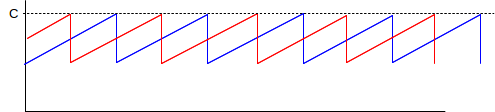Both connections have a maximum cwnd of C. When Connection 1 experiences a loss, Connection 2 will have cwnd = 75% of C, and vice-versa.

(a). What is the combined transit capacity of the paths, in terms of C?
(b). Find the bottleneck-link utilization. Hint: it should be at least 85%.